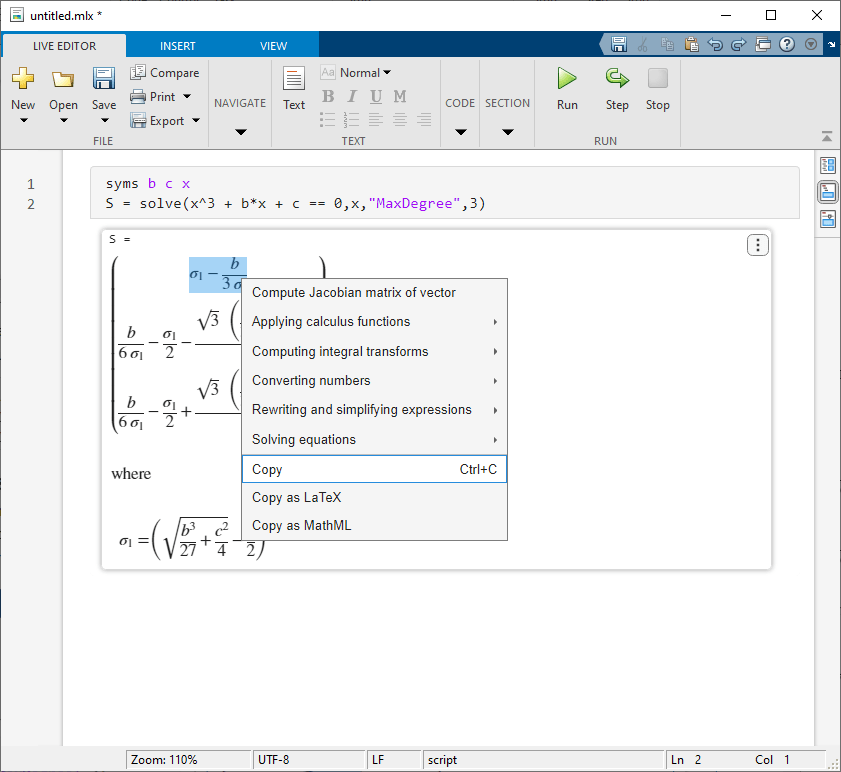# Copy and Paste Symbolic Output in Live Editor

This example shows how to copy symbolic output and paste it as MATLAB code or equation typeset in the MATLAB® Live Editor. To demonstrate this capability, this example uses a cubic (third-degree) polynomial.

### Copy Output and Paste as MATLAB Code

Solve the cubic polynomial ${x}^{3}+bx+c=0$. The solutions are displayed in terms of the abbreviated expression ${\sigma }_{1}$.

```syms b c x S = solve(x^3 + b*x + c == 0,x,'MaxDegree',3)```
```S =  ```

Right-click the symbolic output. Select Copy Output to copy the symbolic expressions that represent the roots of the cubic polynomial.Insert code in the live script and assign the polynomial roots to the variable `Sol`. Then paste the output as MATLAB code using Ctrl + V (or right-click and select Paste). Pasting the output as MATLAB code automatically expands the abbreviated expression.

```Sol = [(sqrt(b^3/27 + c^2/4) - c/2)^sym(1/3) - b/(3*(sqrt(b^3/27 + c^2/4) - c/2)^sym(1/3)); b/(6*(sqrt(b^3/27 + c^2/4) - c/2)^sym(1/3)) - (sqrt(b^3/27 + c^2/4) - c/2)^sym(1/3)/2 - (sqrt(3)*(b/(3*(sqrt(b^3/27 + c^2/4) - c/2)^sym(1/3)) + (sqrt(b^3/27 + c^2/4) - c/2)^sym(1/3))*sym(1i))/2; b/(6*(sqrt(b^3/27 + c^2/4) - c/2)^sym(1/3)) - (sqrt(b^3/27 + c^2/4) - c/2)^sym(1/3)/2 + (sqrt(3)*(b/(3*(sqrt(b^3/27 + c^2/4) - c/2)^sym(1/3)) + (sqrt(b^3/27 + c^2/4) - c/2)^sym(1/3))*sym(1i))/2]```
```Sol =  ```

### Copy Selected Output and Paste as MATLAB Code

Select the first solution of the cubic polynomial. When selecting a subexpression, you can copy and paste only the subexpression that is on the right side of the equal sign. Right-click the existing selection and choose Copy (Ctrl + C) in the context menu.Insert code in the live script and assign the first root of the polynomial to the variable `S1`. Then paste the output as MATLAB code using Ctrl + V (or right-click and select Paste). Pasting the output as MATLAB code automatically expands the abbreviated expression.

`S1 = (sqrt(b^3/27 + c^2/4) - c/2)^sym(1/3) - b/(3*(sqrt(b^3/27 + c^2/4) - c/2)^sym(1/3))`
```S1 =  ${\left(\sqrt{\frac{{b}^{3}}{27}+\frac{{c}^{2}}{4}}-\frac{c}{2}\right)}^{1/3}-\frac{b}{3 {\left(\sqrt{\frac{{b}^{3}}{27}+\frac{{c}^{2}}{4}}-\frac{c}{2}\right)}^{1/3}}$```

### Copy Selected Output and Paste as Equation Typeset

You can also paste a selection as equation typeset. Select the second solution of the cubic polynomial. Right-click the selection and choose Copy (Ctrl + C) in the context menu.Then paste the selection as equation typeset in the live script using Ctrl + V (or right-click and select Paste). The equation typeset is rendered as an editable equation. Note that when you paste the output as equation typeset, abbreviated expressions are not expanded.

`$\frac{b}{6 {\sigma }_{1}}-\frac{{\sigma }_{1}}{2}-\frac{\sqrt{3} \left(\frac{b}{3 {\sigma }_{1}}+{\sigma }_{1}\right) \mathrm{i}}{2}$`

## Support

#### Mathematical Modeling with Symbolic Math Toolbox

Get examples and videos